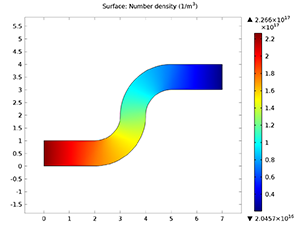# アプリケーションギャラリ

## Molecular Flow Through an S-Bend

Application ID: 14195

This model computes the transmission probability through an s-bend geometry using both the angular coefficient method available in the Free Molecular Flow interface and a Monte Carlo method using the Mathematical Particle Tracing interface. The computed transmission probability by the two methods is in excellent agreement with less than a 1% difference. This model requires the Particle Tracing Module.This model example illustrates applications of this type that would nominally be built using the following products: